# Test: Radiative Heat Transfer Level - 1

## 20 Questions MCQ Test Heat Transfer | Test: Radiative Heat Transfer Level - 1

Description
Attempt Test: Radiative Heat Transfer Level - 1 | 20 questions in 40 minutes | Mock test for Chemical Engineering preparation | Free important questions MCQ to study Heat Transfer for Chemical Engineering Exam | Download free PDF with solutions
QUESTION: 1

### A radiator in a domestic heating system operates at a surface temperature of . Assuming the radiator behaves as a black body, the rate at which it emits the radiant heat per unit area is (Assume σ =5.67 x 10-8 W/m2-k4)

Solution:

As per Stefan Boltzmann law

Eb = Q/A = σ x T4

Eb = 5.67 x 10-8 x (55 + 273)4

= 656.2 W/m2

≃ 0.66 kW/m2

QUESTION: 2

### Sun’s surface at emits maximum spectral power for wavelength of A grey surface at a will Demit maximum spectral power at a wavelength of

Solution:

By Wien’s displacement law,

λm ∝ 1/T

∴ (λm)2/(λm)1 = T1/T2

∴ (λm)2 = (λm)1T1/T2

∴ (λm)2 = 0.5 x 5800/(300 + 273)

(λm)2 = 5.06μm

≃ 5μm

QUESTION: 3

### Two radiating surfaces A1= 6m2 and A2=4m2 have shape factor Then shape factor will be

Solution:

By Reciprocity theorem

A1F12 = A2F21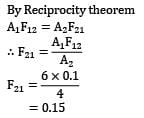= 0.15

QUESTION: 4

What will be the view factor for the geometry as shown in the figure (sphere within a hollow cube)?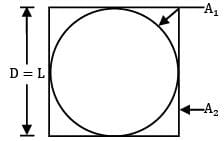Solution: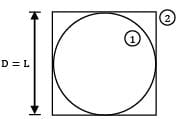1 is a spherical surface

∴ F11 = 0

By summation rule,

F12 + F11 = 1

∴ F12 = 1

By Reciprocity theorem,

A1F12 = A2F21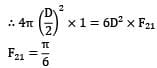QUESTION: 5

Solution:

A radiation shield should reflect back as much radiation as possible. Hence radiation shield should have high reflective power.

QUESTION: 6

For an opaque plane surface at steady state the irradiation, radiosity and emissive power are respectively 20, 12 and . What is the emissivity of the surface?

Solution: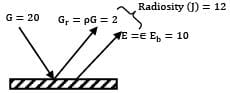J = pG + εEb

For an opaque body

∵ α + ρ = 1

ρ = 1- α

∴ J = (1-α)G + εEb

By kirchoff’s law, α = ε

∴ J = (1-α)G + εEb

12 = (1- ε)20 + 10

ε = 0.9

QUESTION: 7

The wavelength for which the blackbody emissive power is maximum for a temperature of 300 K is

Solution:

By Wien’s displacement law,

∵ λmT = 2898 μm-k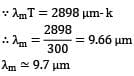λm ≃ 9.7μm

QUESTION: 8

Solar radiation is incident on a semitransparent body at a rate of . If of this incident radiation is reflected back and is transmitted across the body, the absorptivity of the body is

Solution:

G = 500 W/m2 Gr = 150 W/m2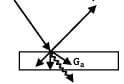Gt = 225 W/m2

By energy conservation principle,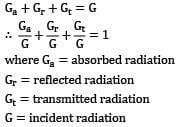Ga+Ge+Gt = G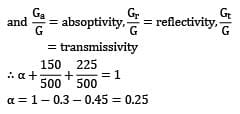= transmissivity

α = 1-0.3 - 0.45 = 0.25

QUESTION: 9

A grey body is defined such that

Solution:
QUESTION: 10

The minimum number of view factors that need to be known to solve a 10-surface enclosure completely, is

Solution:

For n-surface enclosure if nC2 view factors are known directly, entire enclosure can be solved. Thus for 10 surfaces, number of view factors that need to be evaluated directly will be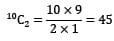QUESTION: 11

The spectral distribution of surface irradiation is as followsWhat is the total irradiation in kW/m2 ?

Solution: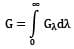i.e. it is equal to area under the curve Gλ Vs λ Hence,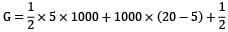x (25-20) x 1000

∴ G = 20000 W/m2

I.e. G = 20 kW/m2

QUESTION: 12

Consider two infinitely long blackbody concentric cylinders with a diameter ratio . The shape factor of the inner surface of outer cylinder with respect to itself will be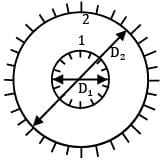Solution: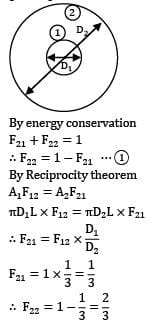By Energy conservation

F12 + F22 =1

∴ F22 = 1-F21 ….①

By Reciprocity theorem

A1F12 = A2F21

πD1L x F12 = πD2L x F21QUESTION: 13

A large spherical enclosure has a small opening. The rate of emission of radiative flux through this opening is . The temperature at the inner surface of the sphere will be about (assume Stefan Boltzmann constant σ = 5.67 x 10-8 W/m2K4)

Solution:

A small opening in a large spherical enclosure behaves as a black surface

∴Eb = σT4

7350 = 5.67 x 10-8 x T4

T = 600K

I.e. T = 327oC

QUESTION: 14

Consider monochromatic emissive power (Eλ) Vs Wavelength(λ) of a black and grey surface both at same temperature. The ratio AB/AC is given as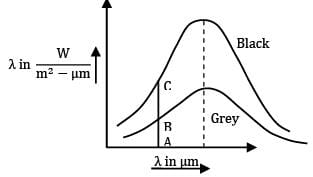Solution:

AB & AC are monochromatic emissive power of grey and black surfaces for same wavelength. Hence, ratio is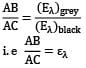which is monochromatic emissivity of grey surface.

QUESTION: 15

If the temperature of a solid surface changes from then its emissive power will increases in the ratio of

Solution:

By Stefan Boltzmann law

E ∝ T4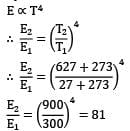QUESTION: 16

Consider a hemispherical furnace. The view factor of it’s roof (hemisphere) with respect to itself is __________.

Solution: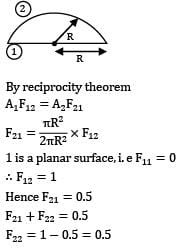By reciprocity theorem

A1F12 = A2F121 is a planar surface, i.e F11 = 0

∴ F12 = 1

Hence F21 = 0.5

F21 + F22 = 0.5

F22 + 1-0.5 = 0.5

QUESTION: 17

Fraction of radiative energy leaving one surface that strikes the other surface is called

Solution:
QUESTION: 18

Ice is very close to a

Solution:

The absorptivity of ice is 0.985, hence ice is very close to a black body

QUESTION: 19

For a grey diffuse surface with emissivity ε and temperature T, the intensity of emitted radiation is given as __________. ( is StefanBoltzmann constant.)

Solution:

For a diffuse surface, intensity of emitted radiation (Ie) is same in all directions and is given as

Ie = E/π

Where E is total emissive power

Therefore

Ie = εσT4

QUESTION: 20

Solar radiation of falls on a grey opaque surface at steady state. The surface has a temperature of and emissivity of 0.8. Find radiosity from the surface?

Solution: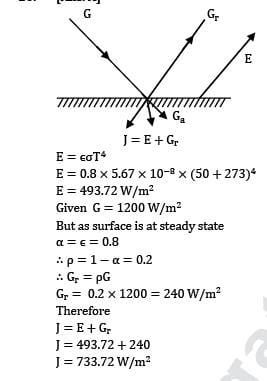J = E+Gr

E = εσT4

E = 0.8 x 5.67 x 10-8 x (50 + 273)4

E = 493.72 W/m2

Given G = 1200 W/m2

But as surface is at steady state

α = ε = 0.8

∴ ρ = 1 -α = 0.2

∴ Gr = ρG

Gr = 0.2 x 1200 = 240 W/m2

Therefore

J = E+Gr

J = 493.72 + 240

J = 733.72 W/m2Use Code STAYHOME200 and get INR 200 additional OFF Use Coupon Code